An Overview of Mixer Classes

## System Components

The chemical reactor is the primary component of the system. We also need source and sink classes to enclose the system. Because both the reactor and the sink class have the same calculated Concentration attribute, we modeled a common superclass, Base.Container, to demonstrate attribute inheritance. That is, the Concentration attribute defined in the Base.Container class are taken by both Reactor and Sink subclasses. Class Base.Container.Reactor has its own referential attributes for setting up the linear equations. The code to establish the constant matrix for the linear equations are primarily in this class.

Classes Base.Source and Base.Source.Variable are not derived from the Base.Container class because the Concentration in the source classes is an input attribute, not a calculated result. Class Link.Pipe contains a Flow Rate attribute, which is entered by the user before a simulation run and remain constant during the simulation run.

## The Solver

The solver is coded in Class Collection.Solver. It contain object references to a reactor table, a constant matrix, an inverse matrix, and a scratch matrix. After the initialization stage of a simulation run, in which the constant matrix is loaded by each of the reactors, a solution routine is called according to the Solver Type decided by the user. The Solver Type is currently implemented globally in the simulation control class. It can, however, be locally defined in Class Collection.Solver. To define an attribute locally or globally depends upon whether we want it to be accessible in the classes other than Class Solver. Other reference classes, Matrix.Calculation and Table.Reactor, are self-explanatory. Consult each class section for more details.

In addition to the simulation control classes, there are nine classes defined in the Schema Definition. We intentionally did so because it can then be loaded into SansGUI Student Edition, which has a limit of 10 developer defined classes, excluding SansGUI intrinsic classes and simulation control classes.

 Icon Class Description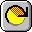SimControl.Cycle.Mixer Simulation control class containing run-time parameters.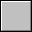Base.Container Abstract class containing common attributes of all subclasses.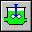Base.Container.Reactor Derived component class to model chemical mixers/reactors.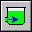Base.Container.Sink Derived component class to model sinks with unlimited capacity.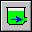Base.Source Component class to model constant sources with unlimited supply.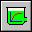Base.Source.Variable Derived component class to model variable sources with a step function.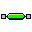Link.Pipe Link component class for connecting Reactors, Sources, and Sinks.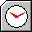Collection.Solver Class in which the primary solvers reside.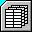Matrix.Calculation Matrices used in the calculations.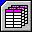Table.Reactor For a table of right-hand-side (r-h-s) constants of the simultaneous equations and the solution vector.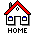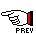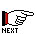Mixer Example for SansGUI Version 1.0